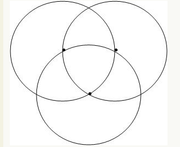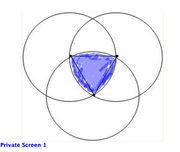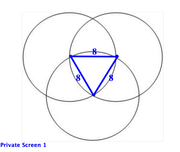• NEW! FREE Beat The GMAT QuizzesHundreds of Questions Highly Detailed Reporting Expert Explanations
• 7 CATs FREE!
If you earn 100 Forum Points

Engage in the Beat The GMAT forums to earn
100 points for $49 worth of Veritas practice GMATs FREEVERITAS PRACTICE GMAT EXAMS Earn 10 Points Per Post Earn 10 Points Per Thanks Earn 10 Points Per Upvote Three congruent circles overlap in such a way that each circ tagged by: Anaira Mitch This topic has 3 expert replies and 2 member replies Three congruent circles overlap in such a way that each circTimer 00:00 Your Answer A B C D E Global Stats Difficult Three congruent circles overlap in such a way that each circle intersects the centers of both of the other circles, as shown below. If the radius of each of the circles is 8, what is the area of the central section where all three circles overlap? A. 16sqrt3 B. 32(pie - sqrt3) C. 16(pie + sqrt3) D. 32 pie E. 32(pie + sqrt3)GMAT/MBA Expert GMAT InstructorJoined 25 May 2010 Posted: 15362 messages Followed by: 1866 members Upvotes: 13060 GMAT Score: 790 Anaira Mitch wrote: The circle is the circumscribed circle of an equilateral triangle. The statement above is incorrect.The shaded region encompassing the equilateral triangle is not a circle. _________________ Mitch Hunt Private Tutor for the GMAT and GRE GMATGuruNY@gmail.com If you find one of my posts helpful, please take a moment to click on the "UPVOTE" icon. Available for tutoring in NYC and long-distance. For more information, please email me at GMATGuruNY@gmail.com. Student Review #1 Student Review #2 Student Review #3 Free GMAT Practice Test How can you improve your test score if you don't know your baseline score? Take a free online practice exam. Get started on achieving your dream score today! Sign up now. GMAT/MBA Expert GMAT InstructorJoined 25 May 2010 Posted: 15362 messages Followed by: 1866 members Upvotes: 13060 GMAT Score: 790 Anaira Mitch wrote: Three congruent circles overlap in such a way that each circle intersects the centers of both of the other circles, as shown below. If the radius of each of the circles is 8, what is the area of the central section where all three circles overlap? A. 16sqrt3 B. 32(pie - sqrt3) C. 16(pie + sqrt3) D. 32 pie E. 32(pie + sqrt3) Area of an equilateral triangle = (s²/4)√3. √3 ≈ 1.7.Each circle has a radius of 8, yielding the equilateral triangle above. Area of the equilateral triangle = (8²/4)√3 = 16√3 ≈ (16)(1.7) = 27. The three circles form an overlap that extends a little beyond the equilateral triangle. Thus, the area of the overlap must be a little more than 16√3 ≈ 27. Eliminate A, since the overlap must have an area greater than 16√3. Of the four remaining answer choices, only B yields a value a little more than 27: 32(π - √3) ≈ 32(3 - 1.7) = 32*1.3 ≈ 41. The correct answer is B. _________________ Mitch Hunt Private Tutor for the GMAT and GRE GMATGuruNY@gmail.com If you find one of my posts helpful, please take a moment to click on the "UPVOTE" icon. Available for tutoring in NYC and long-distance. For more information, please email me at GMATGuruNY@gmail.com. Student Review #1 Student Review #2 Student Review #3 Free GMAT Practice Test How can you improve your test score if you don't know your baseline score? Take a free online practice exam. Get started on achieving your dream score today! Sign up now. Master | Next Rank: 500 PostsJoined 26 Oct 2016 Posted: 235 messages Followed by: 5 members Upvotes: 3 My Approach: The circle is the circumscribed circle of an equilateral triangle. Try to find median of Equilateral triangle( median=altitude=bisector). median square + half side square= full side square median square=64-16=48 median= 4 underoot 3 no,we knowinan equilateral triangle the centroid is divided in ratio of 2/3. therefore radius ofcircumscribed circle is 2/3 of 4 underroot 3 r=8 / underroot 3 Now area of that circle= pie*64/3 which is not an option. GMAT/MBA Expert GMAT InstructorJoined 09 Oct 2010 Posted: 1449 messages Followed by: 32 members Upvotes: 59 Anaira Mitch wrote: Three congruent circles overlap in such a way that each circle intersects the centers of both of the other circles, as shown below. If the radius of each of the circles is 8, what is the area of the central section where all three circles overlap? A. 16sqrt3 B. 32(pi - sqrt3) C. 16(pi + sqrt3) D. 32 pi E. 32(pi + sqrt3)$$? = {S_{\Delta {\rm{equil}}}} + \,\,3 \cdot \,{S_{{\rm{blue}}}}$$ $${S_{\Delta {\rm{equil}}}} = {{{r^{\,2}}\sqrt 3 } \over 4}\,\,\,\mathop = \limits^{r\, = \,8} \,\,16\sqrt 3$$ $${S_{{\rm{blue}}}} = {{60} \over {360}}\left( {\pi \cdot {8^2}} \right) - {S_{\Delta {\rm{equil}}}} = {{\pi \cdot {8^2}} \over 6} - \,16\sqrt 3 = 8\left( {{{4\pi } \over 3} - 2\sqrt 3 } \right)\,\,\,\,\,\,\, \Rightarrow \,\,\,\,\,\,\,\,3 \cdot \,{S_{{\rm{blue}}}} = 8\left( {4\pi - 6\sqrt 3 } \right)$$ $$? = \,\,16\sqrt 3 + 8\left( {4\pi - 6\sqrt 3 } \right) = 32\left( {\pi - \sqrt 3 } \right)\,\,\,\,\,\,\, \Rightarrow \,\,\,\,\,\,\,\left( {\rm{B}} \right)$$ This solution follows the notations and rationale taught in the GMATH method. Regards, Fabio. _________________ Fabio Skilnik :: GMATH method creator ( Math for the GMAT) English-speakers :: https://www.gmath.net Portuguese-speakers :: https://www.gmath.com.brMaster | Next Rank: 500 PostsJoined 26 Oct 2016 Posted: 235 messages Followed by: 5 members Upvotes: 3 GMATGuruNY wrote: Anaira Mitch wrote: Three congruent circles overlap in such a way that each circle intersects the centers of both of the other circles, as shown below. If the radius of each of the circles is 8, what is the area of the central section where all three circles overlap? A. 16sqrt3 B. 32(pie - sqrt3) C. 16(pie + sqrt3) D. 32 pie E. 32(pie + sqrt3) Area of an equilateral triangle = (s²/4)√3. √3 ≈ 1.7.Each circle has a radius of 8, yielding the equilateral triangle above. Area of the equilateral triangle = (8²/4)√3 = 16√3 ≈ (16)(1.7) = 27. The three circles form an overlap that extends a little beyond the equilateral triangle. Thus, the area of the overlap must be a little more than 16√3 ≈ 27. Eliminate A, since the overlap must have an area greater than 16√3. Of the four remaining answer choices, only B yields a value a little more than 27: 32(π - √3) ≈ 32(3 - 1.7) = 32*1.3 ≈ 41. The correct answer is B. Hello Mitch, Can you tell me what went wrong with my approach? •Free Veritas GMAT Class Experience Lesson 1 Live Free Available with Beat the GMAT members only code •5 Day FREE Trial Study Smarter, Not Harder Available with Beat the GMAT members only code •Free Trial & Practice Exam BEAT THE GMAT EXCLUSIVE Available with Beat the GMAT members only code •Magoosh Study with Magoosh GMAT prep Available with Beat the GMAT members only code •Free Practice Test & Review How would you score if you took the GMAT Available with Beat the GMAT members only code •Get 300+ Practice Questions 25 Video lessons and 6 Webinars for FREE Available with Beat the GMAT members only code •1 Hour Free BEAT THE GMAT EXCLUSIVE Available with Beat the GMAT members only code •5-Day Free Trial 5-day free, full-access trial TTP Quant Available with Beat the GMAT members only code •FREE GMAT Exam Know how you'd score today for$0

Available with Beat the GMAT members only code

•Award-winning private GMAT tutoring
Register now and save up to \$200

Available with Beat the GMAT members only code

Top First Responders*

1Brent@GMATPrepNow 41 first replies
2Ian Stewart 37 first replies
3Jay@ManhattanReview 32 first replies
4GMATGuruNY 26 first replies
5Scott@TargetTestPrep 14 first replies
* Only counts replies to topics started in last 30 days
See More Top Beat The GMAT Members

Most Active Experts

1Scott@TargetTestPrep

Target Test Prep

199 posts
2Max@Math Revolution

Math Revolution

92 posts
3Brent@GMATPrepNow

GMAT Prep Now Teacher

74 posts
4GMATGuruNY

The Princeton Review Teacher

45 posts
5Ian Stewart

GMATiX Teacher

43 posts
See More Top Beat The GMAT Experts